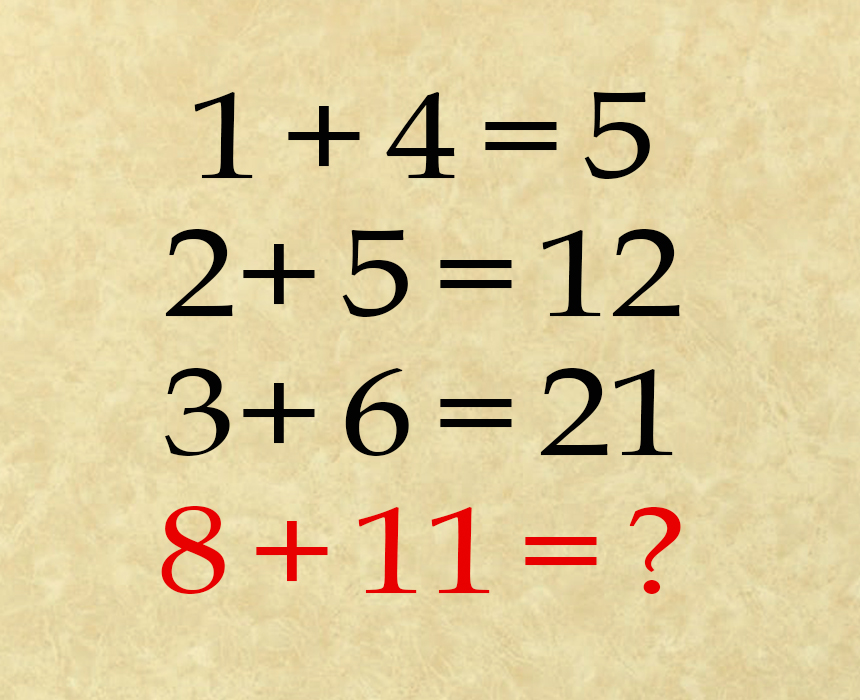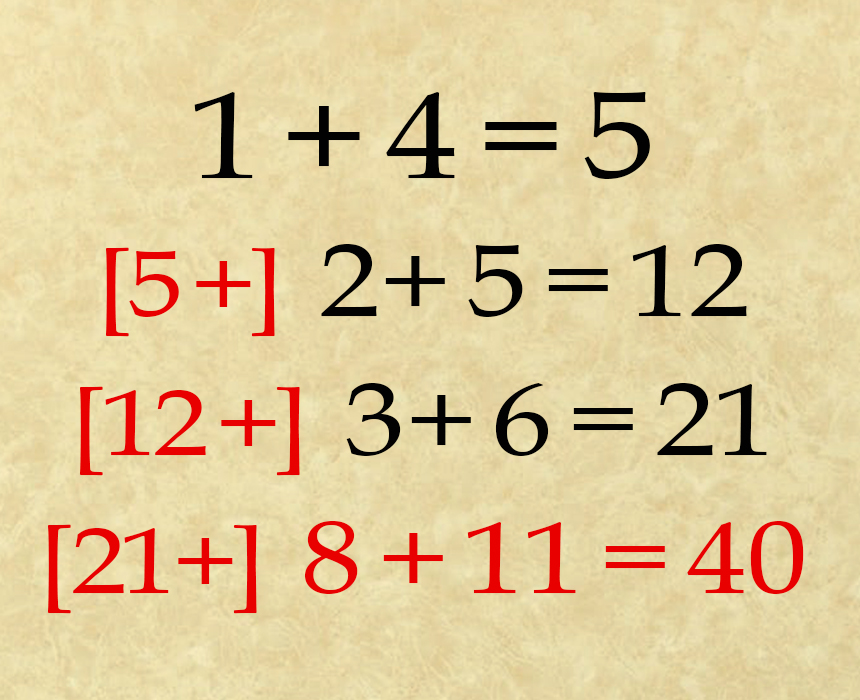# Can you solve this tough number puzzle? It’s leaving so many people confused

We all need to give our brains a work out sometimes with something we wouldn’t normally do. This number puzzle isn’t the norm for everyone but having a go at it will certainly give your gray matter the work out it needs. It’s made so many people stop and stare and yet others get it straight away. Can you solve it and if so how fast? My friend from Michigan solved it in 20 seconds.

I first looked at this puzzle and thought the answer has to be 19 and the other two equations above it are just wrong. I was completely stumped. But, there’s more math to these number sentences than meets the eye. Can you work it out? The first number sentence is obvious: 1 + 4 is definitely 5 but then the next two lines don’t follow suit.

In working out the next two number sentences and why the answer is what it is you will certainly ace the last sentence.If you insist on the missing number being 19, we’ll take it. It’s a trick answer and shows you can think outside of the box but there is another answer.

We’ve established the first answer is obvious but following that rule: 2 + 5 = 12 and 3 + 6 = 21, do not follow the same pattern.

Take the answer to the previous number equation and add it to the number of the next equation.In the case of the first equation, there is no previous equation, and so you would add zero to the first equation (0 + 1 + 4), which gives you 5. The same pattern works for the second and third equations. Apply this rule to the last equation, and you will get the solution. The answer to the puzzle is 40! Did you get it right? It’s easy when you know how. Share this number puzzle with your friends and family and give them a brain workout today.